# Martin Orr's Blog

## Dual abelian varieties over the complex numbers

Posted by Martin Orr on Tuesday, 26 April 2011 at 12:35

In this post I will define dual abelian varieties over the complex numbers. The motivation is that polarisations can be interpreted as isogenies from an abelian variety to its dual. For the moment, all this is tied to Hodge structures so only works over the complex numbers, but this is the view of polarisations which will we will generalise later to other fields.

### Chow's Theorem

I left out a bit in the previous post - I sketched a proof that a polarisable complex torus is projective in the sense that it has a holomorphic embedding in projective space, but in order to show that it is an abelian variety we need to know that the image of this embedding is algebraic. Fortunately, this is automatically true due to the following theorem of complex geometry.

Chow's Theorem. A closed analytic submanifold of complex projective space is an algebraic variety.

A related theorem says that holomorphic maps between complex projective varieties are automatically algebraic morphisms. Furthermore morphisms of complex tori biject with morphisms of their Hodge structures. So we conclude that the following categories are equivalent:Another theorem in the same vein says that holomorphic invertible sheaves on a complex projective variety are algebraic.

###-dual Hodge structures

Recall that we defined a polarisation of anHodge structure to be a certain kind of bilinear form. A bilinear form on a vector spaceis equivalent to a linear map fromto its dual vector space. We would like to similarly define a notion of dual for Hodge structures, so that a polarisation can be viewed as a morphism from a Hodge structure to its dual.

What we are about to define is not what is usually called the dual of a Hodge structure. In the usual terminology, the dual of anHodge structure is anHodge structure. But to stay inside the above equivalence of categories, we need to work only withHodge structures. For want of a better name, I shall call the object we are about to define the-dual of a Hodge structure.

Letbe an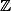-Hodge structure, with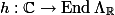defining the complex structure on.

The-module underlying the-dual Hodge structureis of courseThe complex structure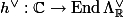is a little less obvious. Recall that one of the conditions for a symplectic form onto be a polarisation is that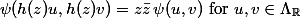or equivalentlyBecause of this conjugation which happens when we movebetween the left and right arguments of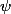, we define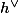to be the conjugate of the most obvious thing: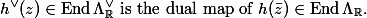Alternatively, in terms of the decomposition, we are definingand likewise for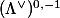.

With this definition, bilinear formsonsatisfying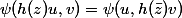biject with morphisms of Hodge structuresviaAs with any duality,is a contravariant functor from the category of-Hodge structures to itself, andis naturally isomorphic to, by the map sendingto "evaluate at". (However when we interpret the dual variety geometrically, it turns out to be better to use the isomorphismsendingto "evaluate at". This is at least partially justified by the fact that we are working with symplectic pairings.)

### Dual abelian varieties

Let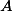be an abelian variety over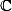anditsHodge structure. The dual abelian variety ofis defined to be the abelian variety associated to the-dual Hodge structure. In order for this definition to make sense, we need to check thatis polarisable.

Choose a polarisationof, and letbe the associated morphism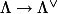. Because the Hermitian form ofis positive definite,is a nondegenerate symplectic form. Henceis an isomorphism of vector spaces. However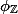is injective but not necessarily surjective -- it has a finite cokernel.

(A morphism of Hodge structureswhich becomes an isomorphism when restricted to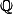-Hodge structures is called an isogeny of Hodge structures, because ifandare polarisable then these are precisely the morphisms which correspond to isogenies of the associated abelian varieties.)

Letbe the index ofin. For any, there is a uniquesuch that. We define a symplectic formbyBecausewas a polarisation, so is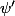. Sois polarisable, and the complex torusis isomorphic to an abelian variety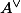.

The above proof tells us that an abelian varietyis always isogenous to its dual -- each polarisation ofgives rise to an isogeny. Howeverandare not always isomorphic. A polarisation whose associated isogeny is an isomorphism is called a principal polarisation.

You can show that, given a free-modulewith a nondegenerate symplectic form, there is a submodule of finite indexsuch that the restriction oftoinduces an isomorphism. Hence every complex abelian variety is isogenous to a principally polarisable abelian variety.

In the case of elliptic curves, every elliptic curve has a unique principal polarisation. This is because the group of symplectic forms on a free-module of rank 2 is isomorphic to. There are two possible isomorphisms, one of which makes positive integers correspond to polarisations. The symplectic form which corresponds tounder this isomorphism is the unique principal polarisation.

1. Dual abelian varieties and line bundles From Martin's Blog

The definition I gave last time of dual abelian varieties was very much dependent on complex analytic methods. In this post I will explain how dual varieties can be interpreted geometrically: the points of correspond to a certain group of line bun...

2. Line bundles and morphisms to the dual variety From Martin's Blog

Over the complex numbers, the dual of an abelian variety is defined to have a Hodge structure dual to that of . Hence morphisms can be interpreted as bilinear forms on the Hodge structure of . Of particular importance are the morphisms correspondi...

3. Weil pairings: definition From Martin's Blog

Recall that for an abelian variety over the complex numbers, is dual to (this is built in to the analytic definition of ). Since , this tells us that is dual to (as -modules). We would like to show that this is true over other fields as well, whic...